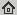# iPiasco: Inertial Proximal Algorithm for strongly convex Optimization

Journal of Mathematical Imaging and Vision, 53(2): 171-181, Oct 2015
Abstract: In this paper, we present a forward--backward splitting algorithm with additional inertial term for solving a strongly convex optimization problem of a certain type. The strongly convex objective function is assumed to be a sum of a non-smooth convex and a smooth convex function. This additional knowledge is used for deriving a worst-case convergence rate for the proposed algorithm. It is proved to be an optimal algorithm with linear rate of convergence. For certain problems this linear rate of convergence is better than the provably optimal worst-case rate of convergence for smooth strongly convex functions. We demonstrate the efficiency of the proposed algorithm in numerical experiments and examples from image processing.

## BibTex reference

```@Article{OB15,
author       = "P. Ochs and T. Brox and T. Pock",
title        = "iPiasco: Inertial Proximal Algorithm for strongly convex Optimization",
journal      = "Journal of Mathematical Imaging and Vision",
number       = "2",
volume       = "53",
pages        = "171-181",
month        = "Oct",
year         = "2015",
url          = "http://lmb.informatik.uni-freiburg.de/Publications/2015/OB15"
}
```